# How to Sum for Cell Contains Formula Only in Excel

Sometimes values are created by formulas in cells. If we want to sum values which are created by formulas from a range, but some values which are hardcoded also list in the same range, how can we filter out matched data and only sum the filtered values correctly? By the way, if we want to sum cells that contains formulas, how can we do? Actually, we can apply ISFORMULA function to help us to solve our problem, it can return true of false if call contains a formula or not.

In this article, we will show you the method of “SUM for Cells that Contains Formula” with the application of ISFORMULA and SUMPRODUCT functions. We will create a simple formula which consists of the two functions.

We will provide a very simple instance in this article, and we will show you the syntax, argument and the usage of ISFORMULA and SUMPRODUCT functions. We will let you know how the formula works step by step clearly. After reading the article, you may know that in which situations we can choose SUMPRODUCT function to sum data.

## EXAMPLE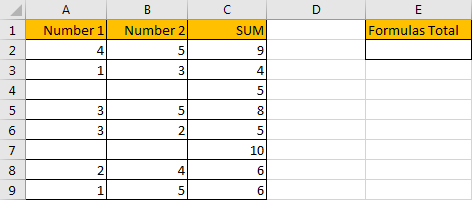In above table, we list the sum of two numbers in SUM column. But for some other numbers in the same column, they are hardcoded for example 5 in C4 and 10 in C7. In E column, we want to sum numbers which are created by formula. If we want to sum numbers ignore the hardcoded numbers, we need the help of ISFORMULA function, it can distinguish if number is generated by a formula or not. Then after filtering numbers, we can apply SUMPRODUCT function to sum filtered numbers.

## FORMULA – APPLY SUMPRODUCT FUNCTION

Step 1: In E2, enter the formula

`=SUMPRODUCT(C2:C9*ISFORMULA(C2:C9))`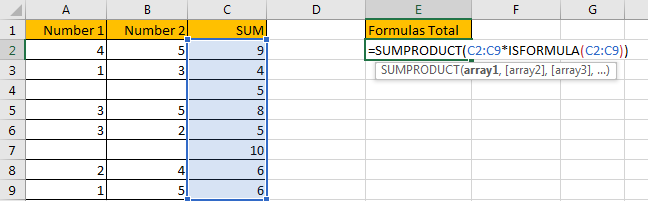Step 2: Press Enter after typing the formula.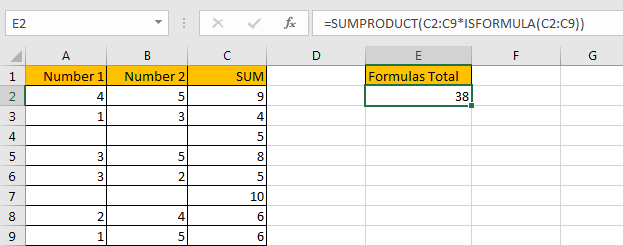We can see that the result is 38, it equals to 9+4+8+5+6+6=38. The formula works correctly.

## FUNCTION INTRODUCTION

SUMPRODUCT function can be seen as SUM+PRODUCT.

For SUMPRODUCT function, the syntax is:

`=SUMPRODUCT(array1,array2,array3, ...)`

For example, enter =SUMPRODUCT({1,2},{2,3}) in any cell, the we can get value 8, it equals to 1*2+2*3=8. You can also enter =SUMPRODUCT({1,2}*{2,3}), you can get the same result. The argument array can be a real array {1,2,3} for example or a range reference like C2:C7.

If there is only array in the formula, SUMPRODUCT will sum the numbers in the array.

Besides, SUMPRODUCT function allows entering texts, logical operators and expressions like (product=cap), texts should be enclosed into ().

ISFORMULA function can return True if cell contains a formula, or if a number is created by a formula; if returns False, that means this cell doesn’t contain any formula.

For ISFORMULA function, the syntax is:

`=ISFORMULA (reference)`

## ARGUMENTS EXPLAINATION

In this formula =SUMPRODUCT(C2:C9*ISFORMULA(C2:C9)), we applied two functions.

For ISFORMULA function, reference is C2:C9.

For SUMPRODUCT function, there is only one array argument, it is C2:C9*ISFORMULA(C2:C9).

## HOW THIS FORMULA WORKS

After explaining argument in the formula, let’s start to execute this formula from inside to outside.

First, in the formula bar, we execute ISFORMULA part. Select ISFORMULA(C2:C9), press F9 to get result, we found that an array is returned. True and False are saved in this array.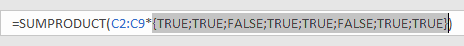Then select C2:C9 in the formula bar, press F9. We can see that values in C2:C9 are expanded in this array.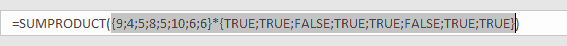Actually, in excel, if the two arrays are multiplied by each other, TRUE will be forced to convert to 1 and FALSE will be converted to 0 in math operation. So, {TRUE;TRUE;FALSE;TRUE;TRUE;FALSE;TRUE;TRUE} will be converted to {1,1,0,1,1,0,1,1} in next step of calculation. At last, this formula can be seen as

`=SUMPRODUCT({9;4;5;8;5;10;6;6}*{1,1,0,1,1,0,1,1}).`

As SUMPRODUCT function can sum products from arrays. That’s why in this case we apply SUMPRODUCT function instead of other sum related functions.

Continue executing the formula. Select {9;4;5;8;5;10;6;6}*{TRUE;TRUE;FALSE;TRUE;TRUE;FALSE;TRUE;TRUE} in the formula bar, press F9. Let’s see if products from above two arrays are displayed.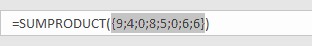As we can see, products are saved a new array {9;4;0;8;5;0;6;6}.

Select =SUMPRODUCT({9;4;0;8;5;0;6;6}) in the formula bar, press F9, 38 is displayed in formula bar. 38 is the final result.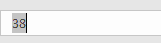1. In this instance, if we want to sum cells which doesn’t contain any cell, we can add NOT before ISFORMULA

Enter the follow formula in Cell E2:

` =SUMPRODUCT(C2:C9*NOT(ISFORMULA(C2:C9)))`

We can get result 15.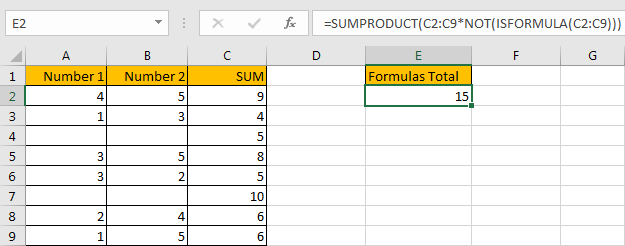NOT function here can return the opposite of the given formula ISFORMULA(C2:C9).

2. In this case we can also use SUM function to replace SUMPRODUCT function. But SUMPRODUCT function can handle arrays directly without entering Ctrl + Shift + Enter, so we often apply SUMPRODUCT function in similar situations.

After applying SUM function, press Ctrl + Shift + Enter simultaneously, we can also get correct result 38.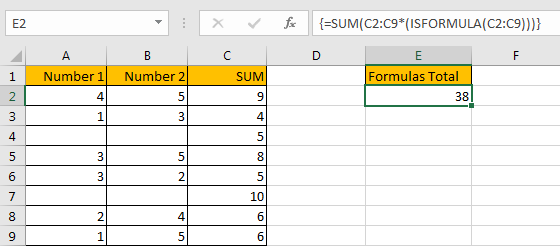## SUMMARY

1. SUMPRODUCT function can handle multiple arrays, and it sum up the products.

2. SUMPRODUCT function allows user defined range, wildcards, logical operators, expressions.

3. ISFORMULA function can return True/False if cell contains a formula or not.

4. NOT function can return the opposite of a given formula or logical operation in excel.

## Related Functions

• Excel SUMPRODUCT function
The Excel SUMPRODUCT function multiplies corresponding components in the given one or more arrays or ranges, and returns the sum of those products.The syntax of the SUMPRODUCT function is as below:= SUMPRODUCT (array1,[array2],…)…
• Excel SUM function
The Excel SUM function will adds all numbers in a range of cells and returns the sum of these values. You can add individual values, cell references or ranges in excel.The syntax of the SUM function is as below:= SUM(number1,[number2],…)
• Excel NOT function
The Excel NOT function returns the opposite of a given logical or Boolean value. For example, if you supplied with the value TRUE, the NOT function will return FALSE; If you supplied with the value FALSE, and the NOT function will TRUE. The syntax of the NOT function is as below:=NOT(logical)…